#### Howdy, Stranger!

We are about to switch to a new forum software. Until then we have removed the registration on this forum.

# how to make gradation ellipse using three color?

edited March 2017

i can make gray gradation ellipse using this way

``````int x;
int y;
int gray;

void setup() {
size(500, 500);
x = width / 2;
y = height / 2;
noStroke();
}

void draw() {
gray = 0;
for(int d = 100; d >= 10; d -= 10) {
fill(gray);
ellipse(x, y, d, d);
gray += 25;
}
}
``````

but how to make gradation ellipse using 3 color (r,g,b)?

i mean, one ellipse with three color gradation

Tagged:

• `fill(r, g, b)`. Use three variables, r, g, and b.

• this way can't make one ellipse with three gradation ;(

• @JJJJJJ
If I understand you correctly you wan't it to look like this?

``````int x;
int y;
int rgb;
float lerpamount;
int middle = #abcdef;
int edge = #fedcba;

void setup() {
size(500, 500);
x = width / 2;
y = height / 2;
noStroke();
}

void draw() {
for(int d = 100; d >= 10; d -= 10) {
lerpamount = norm(d,100,10);
rgb = lerpColor(edge,middle,lerpamount);
fill(rgb);
ellipse(x, y, d, d);
}
}
``````
• oh!!!!!!!!!!! it looks similar!!!! but is this three color ? it looks like two color ellipse to me,. if you have any other way , plz teach me :) thank you so much X)!!

• cab you draw what you want with photoshop?

• edited March 2017

@JJJJJJ

if you have any other way

Yes and I was going to ask you to show a picture how you wanted it like koogs, but then I ran your sketch and figured you wanted it like that but with colors.

Do you want it like A, B or something else?

A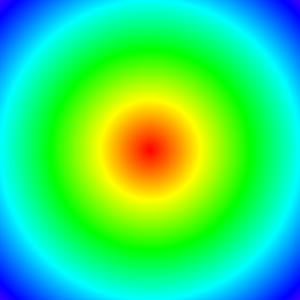B•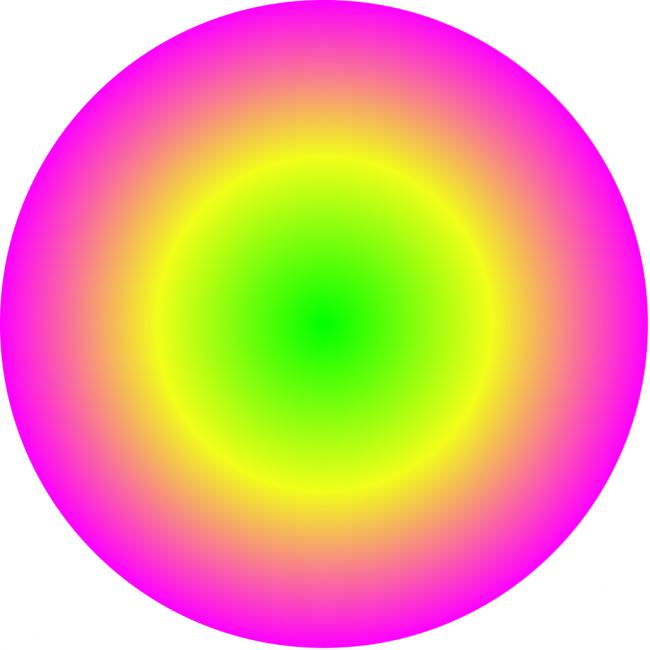@prince_polka @koogs i want like this! one ellipse with three gradation!

• edited March 2017 Answer ✓

as per prince_polka's post but changing the colours halfway through

``````// original by prince polka

int x;
int y;
int rgb;
float lerpamount;
int edge = #ff8888; // pinkish
int halfway = #ffff00;  // yellow
int centre = #00ff00;  // green

void setup() {
size(500, 500);
x = width / 2;
y = height / 2;
noStroke();
}

void draw() {
// outer
for(int d = 200; d >= 100; d -= 10) {
lerpamount = map(d, 200, 100, 0, 1);
rgb = lerpColor(edge, halfway, lerpamount);
fill(rgb);
ellipse(x, y, d, d);
}
// inner
for(int d = 100; d >= 10; d -= 10) {
lerpamount = map(d, 100, 10, 0, 1);
rgb = lerpColor(halfway, centre, lerpamount);
fill(rgb);
ellipse(x, y, d, d);
}
}
``````

I took some time as I rewrote it taking a different approach

``````PImage circle_grad;
int edge = #ff00ff;
int middle = #ffff00;
int center= #00ff00;
int rgb;
void setup(){
size(500,500);
if(dis<2){
if(dis>1){ rgb = lerpColor(middle,edge,dis-1);}
else{ rgb = lerpColor(center,middle,dis);}
} else{ rgb=0x00000000; }
}}
}
``````
• @koogs @prince_polka thank you sooooo much!!!!! XD !!!!!!!

• @JJJJJJJ --

Notice that the built-in function `lerpColor()` takes an amt from 0-1.0 and interpolates a color between them.

Here I've written a new function `lerpColors()` that takes any number of colors (2, 3, 4... 10 etc.) and then uses lerpColor to interpolate a color that appears somewhere between any two items in the list.

``````color lerpColors(float amt, color... colors) {
if(colors.length==1){ return colors; }
float cunit = 1.0/(colors.length-1);
return lerpColor(colors[floor(amt / cunit)], colors[ceil(amt / cunit)], amt%cunit/cunit);
}
``````

This function solves the "3 color" problem, but also the "n color" problem.

Here is a simple demo sketch showing how `lerpColors()` can be used -- either with any number of arguments, or by passing an array of colors as a single argument.

``````/** lerpColors -- interpolates any list of colors rather than just two
* Jeremy Douglass 2017-03-27 Processing 3.2.3
**/

color RED =   color(255,   0,   0);
color GREEN = color(  0, 255,   0);
color BLUE =  color(  0,   0, 255);
color BLACK = color(  0,   0,   0);
color WHITE = color(255, 255, 255);
color[] colors = {RED,GREEN,BLUE,BLACK,WHITE};

void setup() {
size(400, 400);
noStroke();
noLoop();
}

void draw() {
lerpBar(20, 20, 360, 40, BLACK, WHITE);
lerpBar(20, 80, 360, 40, RED, GREEN, BLUE);
lerpBar(20,140, 360, 40, BLUE, GREEN, RED);
lerpBar(20,200, 360, 40, RED, WHITE, RED, BLACK, RED);
lerpBar(20,260, 360, 40, GREEN, WHITE, GREEN, WHITE, GREEN, WHITE, GREEN, WHITE, GREEN);
lerpBar(20,320, 360, 40, colors);
}

void lerpBar(int x, int y, int w, int h, color... colors) {
pushStyle();
for (int i=0; i<w; i++) {
stroke(lerpColors(float(i)/w, colors));
line(x+i, y, x+i, y+h);
}
popStyle();
}

color lerpColors(float amt, color... colors) {
if(colors.length==1){ return colors; }
float cunit = 1.0/(colors.length-1);
return lerpColor(colors[floor(amt / cunit)], colors[ceil(amt / cunit)], amt%cunit/cunit);
}
``````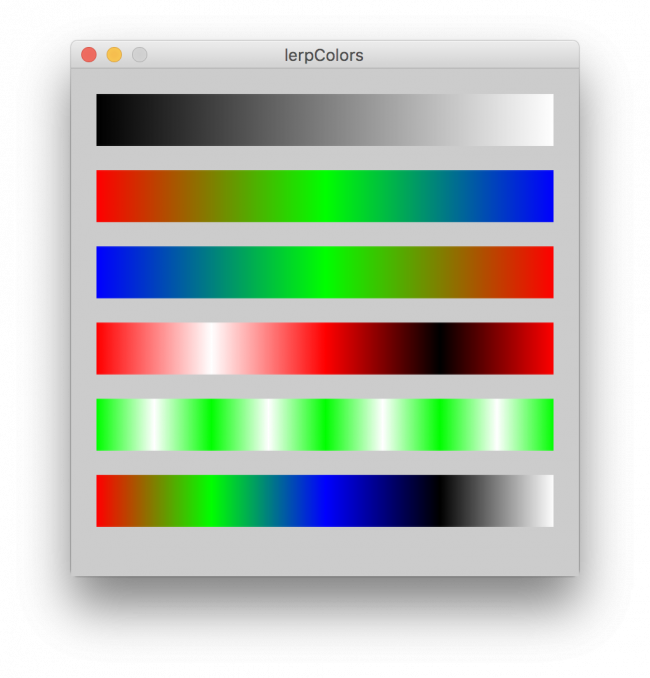• edited March 2017

@Jeremydouglas, that's very cool and easy to use, I made some disco stuff with it

``````PImage g0,g1,g2,g3;
color alpha = 0x00000000;
void setup(){
size(400,400);
}
void keyPressed(){
loop();
}
void draw(){
background(32);
fill(0x0);
rect(100,100,200,200);
noiseSeed(int(random(0xffffff)));
image(g3,200,200,200,200);
image(g2,0,000,200,400);
image(g1,200,0,200,200);
image(g0,100,100,200,200);

noLoop();
}
color lerpColors(float amt, color... colors) {
if(colors.length==1){ return colors; }
float cunit = 1.0/(colors.length-1);
return lerpColor(colors[floor(amt / cunit)], colors[ceil(amt / cunit)], amt%cunit/cunit);
}
int RC(){return int(random(0xffffff))*int(random(256));};
float dia = 200;
PImage foo = createImage(int(dia),int(dia),ARGB);
int rgb=0x0;
float dis=0.5;
boolean square=false;
for (int y=0; y<foo.height; y++){
for (int x=0; x<foo.width; x++){
switch(mode){
case 1:dis = abs(x+y-dia)/dia;break;
case 2:dis = (cos(x*0.1)+PI)/TAU;break;
default:break;
}
if(dis<1 || square){
rgb=lerpColors(dis,colors);
} else if(!square){ rgb=alpha; }
foo.pixels[x+foo.width*y]=rgb;
}}
return foo;
}
``````
• @prince_polka -- Nice use of random color generation, transparency, and noise.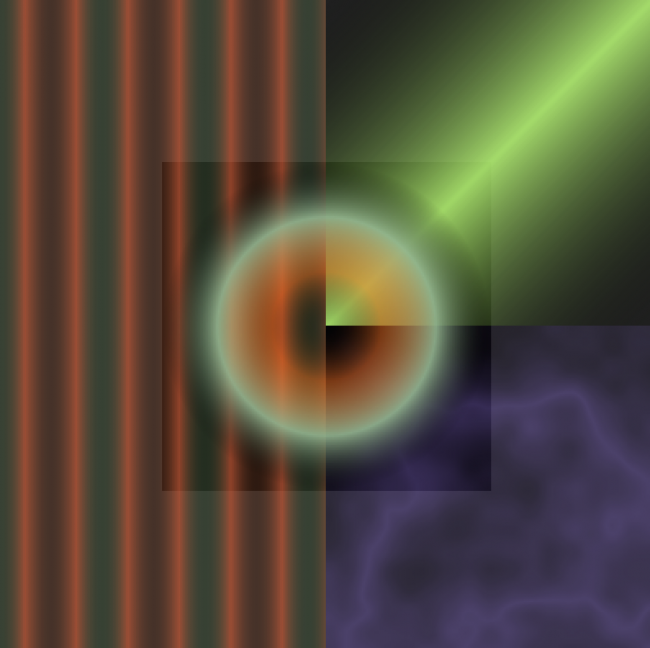I particularly enjoyed playing with the noise mode.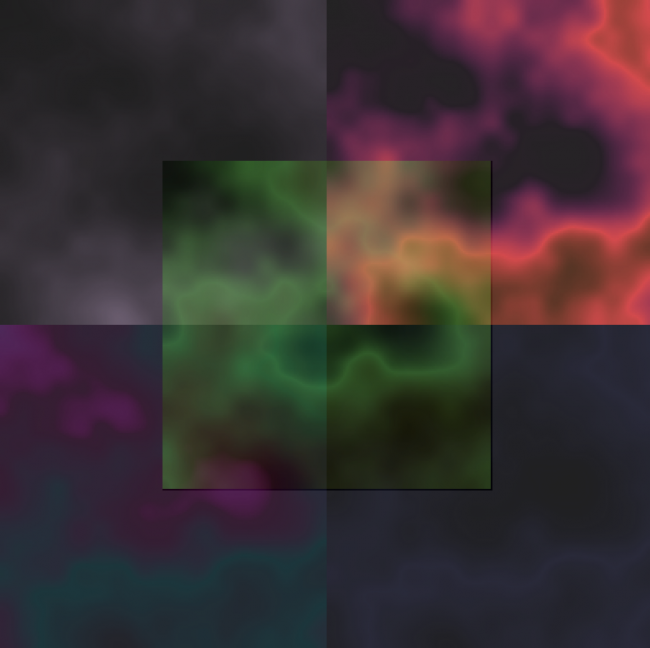• Impressive! Great work you two \m/

Kf

• Beautiful. Some lines of code can produce wonderful effects. ^:)^